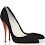## Thursday, September 2, 2010

### Geek Spy: Math Formula Clock

Remember the clock that we showcased a while back that is for the alphabetically inclined? Well, here’s one for math nerds. (Sidenote, why is it that it sounds so much smoother in my head that mathematically inclined people should be nerds rather than geeks? Hmm, a conundrum to ponder over a rainy day methinks.) Anyway, makers of this clock want to ensure that those calculus lessons, math classes haven’t gone to waste. Of course, most of us past a certain age learned to read an analog clock by its angles, so numerals are kinda unnecessary but nice concept. How many do you know? Answers after the cut. Get it for US\$25 here.

1 - Legendre's constant is a mathematical constant occurring in a formula conjectured by Adrien-Marie Legendre to capture the asymptotic behavior of the prime-counting function. Its value is now known to be exactly 1.
2 - A joke in the math world: An infinite number of mathematicians walk into a bar. The first one orders a beer. The second orders half a beer. The third, a quarter of a beer. The bartender says, "You're all idiots," and pours two beers.
3 - A unicode character XML "numeric character reference."
4 - Modular arithmetic, also known as clock arithmetic, is a system of arithmetic for integers, where numbers "wrap around" after they reach a certain value. The modular multiplicative inverse of 2 (mod 7) is the integer /a/ such that 2*/a/ is congruent to 1 modulo 7.
5 - The Golden Mean...reworked a little.
6 - Three factorial (3*2*1=6)
7 - A repeating decimal that is proven to be exactly equal to 7 with Cauchy's Convergence Test.
8 - Graphical representation of binary code.
9 - An example of a base-4 number, which uses the digits 0, 1, 2 and 3 to represent any real number.
10 - A Binomial Coefficient, also known as the choose function. 5 choose 2 is equal to 5! divided by (2!*(5-2)!)
11 A hexadecimal, or base-16, number.1.Haha, very funny, what if you get up in the morning and, still sleepy, can't get the time right! :)

2.3.